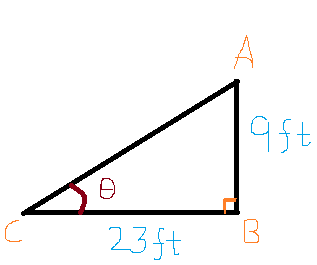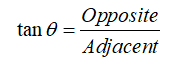# A monster truck drives off a ramp in order to jump onto a row of cars. The ramp has a height of 9 feet and a horizontal length of 23 feet. What is the angle θ of the ramp? (θ is the angle of elevation.) Round your answer to the nearest integer, if necessary.

Question
10 views

A monster truck drives off a ramp in order to jump onto a row of cars. The ramp has a height of 9 feet and a horizontal length of 23 feet. What is the angle θ of the ramp? (θ is the angle of elevation.) Round your answer to the nearest integer, if necessary.

check_circle

Step 1

To Determine: The angle θ of the ramp.

A monster truck drives off a ramp in order to jump onto a row of cars. The ramp has a height of 9 feet and a horizontal length of 23 feet.

Step 2

Given that,

Height of the ramp is 9 feet.

Horizontal length is 23 feet.

Now we will draw a triangle.Step 3

Formula Used:

We know that,...

### Want to see the full answer?

See Solution

#### Want to see this answer and more?

Solutions are written by subject experts who are available 24/7. Questions are typically answered within 1 hour.*

See Solution
*Response times may vary by subject and question.
Tagged in